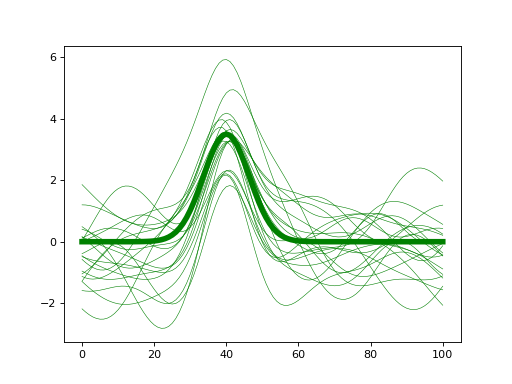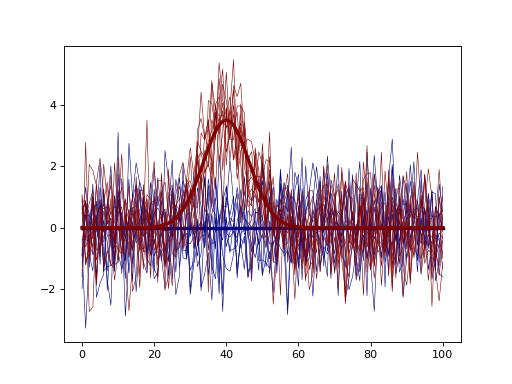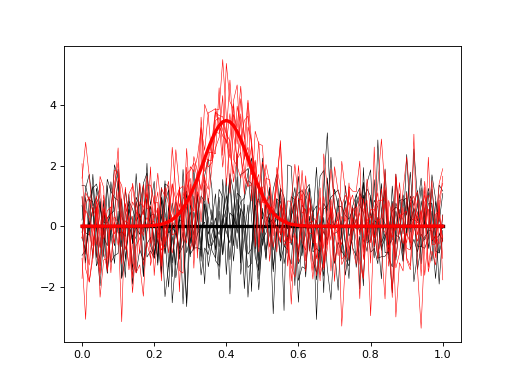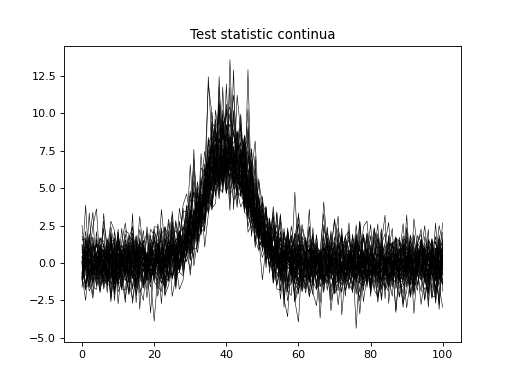# power1d.models¶

High-level classes for assembling data models and simulating experiments.

Below attributes and methods can be distinguished as follows:

• attribute names are followed by “= None”

• method names are followed by “( )” with arguments inside the parentheses, and all methods also have code snippets attached.

## DataSample¶

class `power1d.models.``DataSample`(baseline, signal, noise, J=8, regressor=None)

Data Sample model.

Arguments:

baseline —- a power1d.geom object representing the sample datum

signal —- a power1d.geom object representing the sample signal

noise —- a power1d.noise object representing the sample noise

J —- sample size (int, default 8)

regressor —- an optional regressor that will scale the signal across the J observations (length-J numpy array)

Example:

```from matplotlib import pyplot
import power1d

J        = 20
Q        = 101
baseline = power1d.geom.Null( Q=Q )
signal   = power1d.geom.GaussianPulse( Q=Q, q=40, fwhm=15, amp=3.5 )
noise    = power1d.noise.SmoothGaussian( J=J, Q=Q, mu=0, sigma=1.0, fwhm=20 )
model    = power1d.models.DataSample(baseline, signal, noise, J=J)
pyplot.close('all')
model.plot(color="g", lw=5)
````J`

sample size

`Q`

continuum size

`baseline`

baseline model

`get_baseline`()

Return the DataSample’s baseline object

`get_noise`()

Return the DataSample’s noise object

`get_signal`()

Return the DataSample’s signal object

`hasregressor`

whether or not a regressor has been associated with the data sample

Link noise to another DataSample object so that the linked object’s noise is equivalent to master object’s noise.

`noise`

noise model

`plot`(ax=None, with_noise=True, color='k', lw=3, q=None)

Plot an instantaneous representation of the DataSample

Arguments:

ax —- pyplot axes object (default None)

with_noise —- whether or not to plot the noise (bool, default True)

color —- a matplotlib color for all lines (baseline, signal and noise) (default “k” – black)

lw —- linewidth for the signal (int, default 3)

q —- optional continuum position values (length-Q array of monotonically increasing values) (default None)

Example:

```from matplotlib import pyplot
import power1d

J        = 8
Q        = 101
baseline = power1d.geom.Null( Q=Q )
signal   = power1d.geom.GaussianPulse( Q=Q, q=75, fwhm=15, amp=5.0 )
noise    = power1d.noise.Gaussian( J=J, Q=Q, mu=0, sigma=1.0 )
model    = power1d.models.DataSample(baseline, signal, noise, J=J)
pyplot.close('all')
model.plot(color="b", lw=5)
````random`(subtract_baseline=False)

Generate a random data sample based on the baseline, signal and noise models. The value will be stored in the “value” attribute.

`regressor`

(J,) numpy array representing regressor

`set_baseline`(baseline)

Change the DataSample’s baseline object

Arguments:

baseline —- a power1d.geom object representing the sample datum

`set_noise`(noise)

Change the DataSample’s baseline object

Arguments:

baseline —- a power1d.noise object representing the sample datum

`set_regressor`(x)

Set or change the DataSample’s regressor

Arguments:

x —- a length-J numpy array (float)

`set_sample_size`(J)

Change the sample size

Arguments:

J —- sample size (positive int)

`set_signal`(signal)

Change the DataSample’s signal object

Arguments:

signal —- a power1d.geom object representing the sample signal

`signal`

signal model

`value0`

value in the absence of noise (baseline + signal)

## Experiment¶

class `power1d.models.``Experiment`(data_sample_models, fn)

Experiment model.

Arguments:

data_sample_models —- a list of DataSample objects

fn —- a function for computing the test statistic (must accept a sequence of (J,Q) arrays and return a length-Q array representing the test statistic continuum). Standard test statistic functions are available in power1d.stats

Example:

```from matplotlib import pyplot
import power1d

J        = 10
Q        = 101
baseline = power1d.geom.Null( Q=Q )
signal0  = power1d.geom.Null( Q=Q )
signal1  = power1d.geom.GaussianPulse( Q=Q, q=40, fwhm=15, amp=3.5 )
noise    = power1d.noise.Gaussian( J=J, Q=Q, mu=0, sigma=1.0 )
model0   = power1d.models.DataSample(baseline, signal0, noise, J=J)
model1   = power1d.models.DataSample(baseline, signal1, noise, J=J)
emodel   = power1d.models.Experiment( [model0, model1], fn=power1d.stats.t_2sample )
pyplot.close('all')
emodel.plot()
````Q`

continuum length

`Z`

output test statistic continua

`data_models`

data models

`fn`

test statistic function

`nmodels`

number of data models

`plot`(ax=None, with_noise=True, colors=None, q=None)

Plot an instantaneous representation of the Experiment model.

Arguments:

ax —- pyplot axes object (default None)

with_noise —- whether or not to plot the noise (bool, default True)

colors —- an optional sequence of matplotlib colors, one for each DataSample (default None)

q —- optional continuum position values (length-Q array of monotonically increasing values) (default None)

Example:

```import numpy as np
from matplotlib import pyplot
import power1d

J        = 8
Q        = 101
baseline = power1d.geom.Null( Q=Q )
signal0  = power1d.geom.Null( Q=Q )
signal1  = power1d.geom.GaussianPulse( Q=Q, q=40, fwhm=15, amp=3.5 )
noise    = power1d.noise.Gaussian( J=J, Q=Q, mu=0, sigma=1.0 )
model0   = power1d.models.DataSample(baseline, signal0, noise, J=J)
model1   = power1d.models.DataSample(baseline, signal1, noise, J=J)
emodel   = power1d.models.Experiment( [model0, model1], fn=power1d.stats.t_2sample )
pyplot.close('all')
emodel.plot( colors=("k", "r"), q=np.linspace(0, 1, Q) )
````set_sample_size`(JJ)

Change the sample sizes of the models

Arguments:

J —- sample size (positive int or list of positive int)

`simulate`(iterations=1000, progress_bar=True)

Iteratively simulate a number of experiments.

Calling this method will repeatedly call simulate_single_iteration and will store the resulting continua in the Z attribute; if 1000 iterations are executed then Z will be a (1000,Q) array when simulation is complete.

Arguments:

iterations —- number of iterations to perform (positive int, default 1000)

progress_bar —- whether or not to display a progress bar in the terminal (bool, default True)

Example:

```import numpy as np
from matplotlib import pyplot
import power1d

J        = 8
Q        = 101
baseline = power1d.geom.Null( Q=Q )
signal0  = power1d.geom.Null( Q=Q )
signal1  = power1d.geom.GaussianPulse( Q=Q, q=40, fwhm=15, amp=3.5 )
noise    = power1d.noise.Gaussian( J=J, Q=Q, mu=0, sigma=1.0 )
model0   = power1d.models.DataSample(baseline, signal0, noise, J=J)
model1   = power1d.models.DataSample(baseline, signal1, noise, J=J)
emodel   = power1d.models.Experiment( [model0, model1], fn=power1d.stats.t_2sample )

emodel.simulate(iterations=50, progress_bar=True)
pyplot.close('all')
pyplot.plot( emodel.Z.T, color='k', lw=0.5 )
pyplot.title('Test statistic continua')
````simulate_single_iteration`()

Simulate a single experiment.

Calling this method will generate new data samples, one for each DataSample model, and will then compute and return the test statistic continuum associated with the new data samples.

## ExperimentSimulator¶

class `power1d.models.``ExperimentSimulator`(model0, model1)

Two-experiment simulator.

This is a convenience class for simulating two experiments:

• “null” experiment: represents the null hypothesis

• “effect” experiment: represents the alternative hypothesis

Simulation results will all share the following characteristics:

• Alpha-based critical thresholds are be computed for the “null” experiment

• Power calculations use those thresholds to conducted power analysis on the “effect” experiment.

Arguments:

model0 —- the “null” experiment model (power1d.models.Experiment) model1 —- the “effect” experiment model (power1d.models.Experiment)

Note

If the “null” and “effect” models are identical then power will be alpha (or numerically close to it) by definition.

`Q`

continuum size

`load_simulation_results`(filename)

Arguments:

filename —- full path to saved results file

Outputs:

results —- a results object (power1d.results.SimulationResults)

Note

Simulating experiments with large sample sizes, complex DataSample models and/or a large number of simulation iterations can be computationally demanding. The load_simulation_results method allows you to probe simulation results efficiently, by saving the results to disk following simulation, then subsequently skipping the “simulate” command. In the example below the commands between the “###” flags should be commented after executing “simulate” once.

Example:

```import numpy as np
from matplotlib import pyplot
import power1d

J        = 8
Q        = 101
baseline = power1d.geom.Null( Q=Q )
signal0  = power1d.geom.Null( Q=Q )
signal1  = power1d.geom.GaussianPulse( Q=Q, q=40, fwhm=15, amp=1.2 )
noise    = power1d.noise.Gaussian( J=J, Q=Q, mu=0, sigma=1.0 )
model0   = power1d.models.DataSample(baseline, signal0, noise, J=J)
model1   = power1d.models.DataSample(baseline, signal1, noise, J=J)

emodel0  = power1d.models.Experiment( [model0, model0], fn=power1d.stats.t_2sample )
emodel1  = power1d.models.Experiment( [model0, model1], fn=power1d.stats.t_2sample )

###  COMMANDS BELOW SHOULD BE COMMENTED AFTER EXECUTING ONCE
sim      = power1d.models.ExperimentSimulator(emodel0, emodel1)
results  = sim.simulate(iterations=200, progress_bar=True)
fname    = "/tmp/results.npz"
results.save( fname )
###  COMMENTS ABOVE SHOULD BE COMMENTED AFTER EXECUTING ONCE

#Then the results can be re-loaded:
fname         = "/tmp/results.npz"
pyplot.close('all')
saved_results.plot()
````model0`

“null” experiment model

`model1`

“effect” experiment model

`simulate`(iterations=50, progress_bar=True)

Iteratively simulate a number of experiments.

Calling this method will repeatedly call simulate_single_iteration for both the “null” model and the “effect” model.

Arguments:

iterations —- number of iterations to perform (positive int, default 1000)

progress_bar —- whether or not to display a progress bar in the terminal (bool, default True)

Outputs:

results —- a results object (power1d.results.SimulationResults)

Example:

```import numpy as np
from matplotlib import pyplot
import power1d

J        = 8
Q        = 101
baseline = power1d.geom.Null( Q=Q )
signal0  = power1d.geom.Null( Q=Q )
signal1  = power1d.geom.GaussianPulse( Q=Q, q=40, fwhm=15, amp=1.0 )
noise    = power1d.noise.Gaussian( J=J, Q=Q, mu=0, sigma=0.3 )
model0   = power1d.models.DataSample(baseline, signal0, noise, J=J)
model1   = power1d.models.DataSample(baseline, signal1, noise, J=J)

emodel0  = power1d.models.Experiment( [model0, model0], fn=power1d.stats.t_2sample )
emodel1  = power1d.models.Experiment( [model0, model1], fn=power1d.stats.t_2sample )

sim      = power1d.models.ExperimentSimulator(emodel0, emodel1)
results  = sim.simulate(iterations=200, progress_bar=True)

pyplot.close('all')
results.plot()
```